Lagrange equation

Definition

General form

A Lagrange equation' is a first-order differential equation that is linear in both the dependent and independent variable, but not in terms of the derivative of the dependent variable. Explicitly, if the independent variable is$x$ and the dependent variable is$y$, the Lagrange equation has the form:$\! F(y')x + G(y')y = H(y')$

Normalized for the dependent variable

Starting with the general form, we can solve for$y$ (dividing both sides by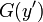$G(y')$) to get an equation of the form: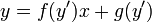$\! y = f(y')x + g(y')$

where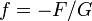$f = -F/G$ and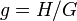$g = H/G$. Note that this process may involve some loss of solutions, since it excludes the possibility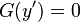$G(y') = 0$. Those solution cases can be considered separately. For the rest of the discussion, we assume that the equation is in the "solved for$y$" form.

Note that the special case where$f$ is the identity map (i.e.,$f(y') = y'$) gives us an equation known as Clairaut's equation. The analysis of Clairaut's equation is slightly different from the general case, so we assume for our discussion that we do not have the Clairaut's equation case. For the Clairaut's equation case, see Clairaut's equation.

Solution method for the non-Clairaut case

We differentiate both sides with respect to$x$ to obtain: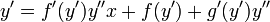$\! y' = f'(y')y''x + f(y') + g'(y')y''$

We now see that the differential equation involves only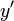$y'$ and higher derivatives, so set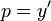$p = y'$ to get:$\! p = f'(p)p'x + f(p) + g'(p)p'$

This becomes: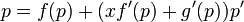$\! p = f(p) + (xf'(p) + g'(p))p'$

so that: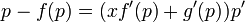$\! p - f(p) = (xf'(p) + g'(p))p'$

We switch the roles of dependent and independent variable, thinking of$x$ as the dependent variable now. We can rewrite the above differential equation as:$\! (p - f(p))\frac{dx}{dp} = xf'(p) + g'(p)$

Rearranging:$\! (p - f(p))\frac{dx}{dp} - f'(p)x = g'(p)$

Now, we separate out the solution possibility$f(p) = p$. For any other solution, we divide by$p - f(p)$ to get a first-order linear differential equation which we can solve for$x$ in terms of$p$. Suppose the general solution is of the form: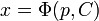$x = \Phi(p,C)$

Then, the overall general solution is given by the following parametric curve:$x = \Phi(p,C), y = f(p)\Phi(p,C) + g(p)$

In addition, there may be special solutions corresponding to the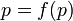$p = f(p)$ case. Specifically, for all$p$ satisfying$p = f(p)$ (hopefully, a discrete set of values), we have straight line solutions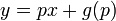$y = px + g(p)$.

Comparison with the Clairaut case

In the Clairaut case, where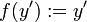$f(y') := y'$, the solution method starts off similarly, but there are some key differences. For the Lagrange equation, the parametric curves form the general solution family and the straight line solutions corresponding to$p = f(p)$ are special solutions (which we may or may not be able to combine with the general solution). In contrast, in the Clairaut case, the general solution is a straight line family and there is a single parametric curve solution that is special (and is the envelope of the straight lines in question).